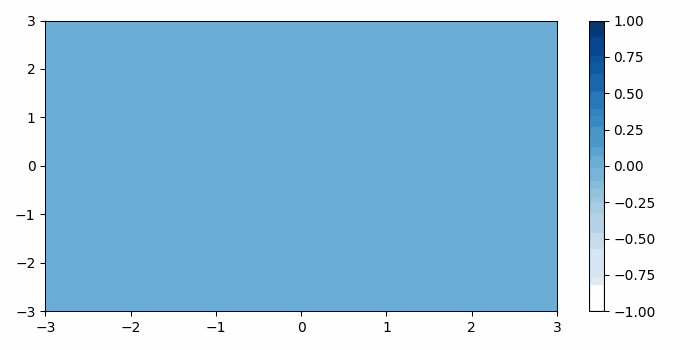# How to animate a pcolormesh in Matplotlib?

To animate pcolormesh in matplotlib, we can take the following steps −

• Create a figure and a set of subplots.

• Create x, y and t data points using numpy.

• Create X3, Y3 and T3, return coordinate matrices from coordinate vectors using meshgrid.

• Create a pseudocolor plot with a non-regular rectangular grid using pcolormesh() method.

• Make a colorbar with colormesh axis.

• Animate pcolormesh using Animation() class method.

• To display the figure, use show() method.

## Example

import numpy as np
from matplotlib import pyplot as plt, animation
plt.rcParams["figure.figsize"] = [7.00, 3.50]
plt.rcParams["figure.autolayout"] = True

fig, ax = plt.subplots()
x = np.linspace(-3, 3, 91)
t = np.linspace(0, 25, 30)
y = np.linspace(-3, 3, 91)
X3, Y3, T3 = np.meshgrid(x, y, t)
sinT3 = np.sin(2 * np.pi * T3 / T3.max(axis=2)[..., np.newaxis])
G = (X3 ** 2 + Y3 ** 2) * sinT3
cax = ax.pcolormesh(x, y, G[:-1, :-1, 0], vmin=-1, vmax=1, cmap='Blues')
fig.colorbar(cax)

def animate(i):
cax.set_array(G[:-1, :-1, i].flatten())

anim = animation.FuncAnimation(fig, animate, interval=100, frames=len(t) - 1)
anim.save('517.gif')
plt.show()

## Output